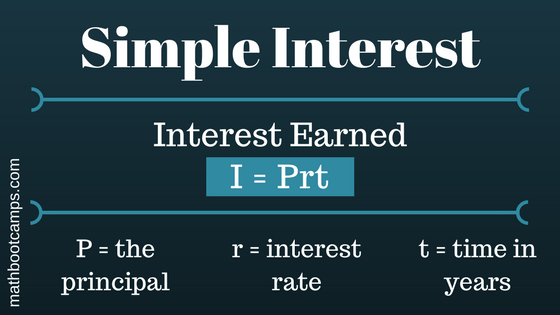# Simple interest formula and examples

Simple interest is when the interest on a loan or investment is calculated only on the amount initially invested or loaned. This is different from compound interest, where interest is calculated on on the initial amount and on any interest earned. As you will see in the examples below, the simple interest formula can be used to calculate the interest earned, the total amount, and other values depending on the problem.

## Examples of finding the interest earned with the simple interest formula

In many simple interest problems, you will be finding the total interest earned over a set period, which is represented as $$I$$. The formula for this is:Let’s use an example to see how this formula works. Remember that in the formula, the principal $$P$$ is the initial amount invested.

A 2-year loan of $500 is made with 4% simple interest. Find the interest earned. ### Solution Always take a moment to identify the values given in the problem. Here we are given: • Time is 2 years: $$t = 2$$ • Initial amount is$500: $$P = 500$$
• The rate is 4%. Write this as a decimal: $$r = 0.04$$

Now apply the formula:

\begin{align}I &= Prt \\ &= 500(0.04)(2) \\ &= \bbox[border: 1px solid black; padding: 2px]{40}\end{align}

### Solution

Before we can apply the formula, we will need to write the time of 4 months in terms of years. Since there are 12 months in a year:

\begin{align}t &= \dfrac{4}{12} \\ &= \dfrac{1}{3}\end{align}

With this adjusted to years, we can now apply the formula with $$P = 1200$$ and $$r = 0.06$$.

\begin{align}I &= Prt \\ &= 1200(0.06)\left(\dfrac{1}{3}\right) \\ &= \bbox[border: 1px solid black; padding: 2px]{24}\end{align}

### Solution

The total amount they will repay is the future value, $$A$$. We are also given that:

• $$t = 8$$
• $$r = 0.075$$
• $$P = 10\,000$$

Using the simple interest formula for future value:

\begin{align}A &= P(1 + rt)\\ &= 10\,000(1 + 0.075(8)) \\ &= \bbox[border: 1px solid black; padding: 2px]{16\,000}\end{align}

Answer: The business will pay back a total of $16,000. This may seem high, but remember that in the context of a loan, interest is really just a fee for borrowing the money. The larger the interest rate and the longer the time period, the more expensive the loan. Also note that you could calculate this by first finding the interest, I = Prt = 10000(0.075(8)) =$6000, and adding it to the principal of \$10000. The final answer is the same using either method.